Skip to content
Related Articles
How to reverse a Colormap using Matplotlib in Python?
• Last Updated : 03 Mar, 2021

Prerequisites: Matplotlib

Matplotlib has many built-in Colormaps. Colormaps are nothing but the dictionary which maps the integer data/numbers into colors. Colormaps are used to differentiate or distinguish the data in a particular plot. The reason to use the colormaps is that it is easier for humans to distinguish the data with respect to other data through the plot having different colors as compared to the numerical values.

Colormaps are classified into four categories depending upon the usage and the requirement are as follows:

• Sequential
• Diverging
• Qualitative
• Miscellaneous

In this article, we are going to reverse the colormap using the Matplotlib library.

Reversing the colormap means reversing the colormap of the plot. For example, if the higher values of the plot are shown with dark blue color and lower values are shown with yellow color then after reversing the colormap the higher values of the plot are shown with yellow color and lower values are shown with dark blue color.

We can reverse the colormap of the plot with the help of two methods:

1. By using the reversed() function to reverse the colormap.
2. By using “_r” at the end of colormap name.

### Step-by-Step Approach:

• Import necessary libraries.
• Create or import dataset for making the plot.
• We can reverse the colormap of the plot by using two methods are discussed above.
• By using the first method:
• First we have to get the colormap using cm.get_cmap(“name_of_cmap”) and let be stored in variable named as “orig_map”.
• Then we have to reverse the original colormap for that we use reversed() function for reversing the colormap we will write orig_map.reversed(), from this we will get reversed colormap let it be stored in a variable named as “reversed”.
• Now, make the scatter plot by passing the values to plot, c for color, and cmap=reversed for getting the reversed color map.
• By using the second method:
• This method is much more simple as compared to the first method, in this method make the scatter plot and by passing the values to plot, c for color just pass the name of colormap by adding _r cmap=”nameofcmap_r” for getting the reversed color map.
• Now give a name to the X and Y axis by using xlabel() and ylabel() function, colorbar for visualizing the mapping of values to colors by using colorbar() function, and give a title to the plot by using title() function provided by matplotlib.
• Now visualize the plot by using show() function provided by matplotlib library.

In this article reversing of colormaps is done by making the Scatter Plots. Let’s do some examples for a better understanding of the topic.

Example 1: Scatter Plot with default colormap without reversing using matplotlib library.

## Python

 `# importing the necessary libraries``import` `matplotlib.pyplot as plt``import` `numpy as np `` ` `# creating the array to plot``x``=``np.arange(``15``)``y``=``[``1``, ``2``, ``3``, ``4``, ``5``, ``6``, ``7``, ``8``, ``9``, ``12``,``   ``15``, ``17``, ``19``, ``21``, ``23``]`` ` `# making the scatter plot for x and y values``# giving color to the plot with respect to y``plt.scatter(x, y, c ``=` `y)`` ` `# naming the x axis and``# y axis using xlabel() and ylabel() function ``plt.xlabel(``"X Axis"``)``plt.ylabel(``"Y Axis"``)`` ` `# visualizing the mapping from values to colors``plt.colorbar()`` ` `# giving title to the plot using plt.title() function``plt.title(``"Scatter Plot with Default colormap"``)`` ` `# visualizing the plot``plt.show()`

Output: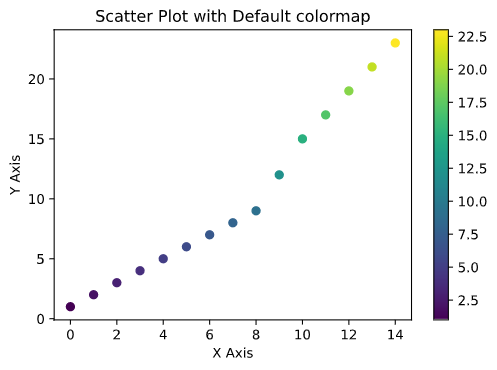This is the scatter plot with default colormap. In this example, while plotting had not passed the cmap=”viridis” parameter because the scatter plot uses viridis colormap as a default colormap. Now let’s reverse the colormap and see how well it looks like.

Example 2: Scatter Plot by reversing the colormap by using reversed() function.

## Python

 `# importing the necessary libraries``import` `matplotlib.pyplot as plt``import` `numpy as np `` ` `# creating the array to plot``x``=``np.arange(``15``)``y``=``[``1``, ``2``, ``3``, ``4``, ``5``, ``6``, ``7``,``   ``8``, ``9``, ``12``, ``15``, ``17``, ``19``, ``21``, ``23``]`` ` `# getting the original colormap using cm.get_cmap() function``orig_map``=``plt.cm.get_cmap(``'viridis'``)`` ` `# reversing the original colormap using reversed() function``reversed_map ``=` `orig_map.``reversed``()`` ` `# making the scatter plot on x and y  ``# giving color to the plot with respect``# to y and passing cmap=reversed_map to reverse the colormap``plt.scatter(x, y, c ``=` `y, cmap ``=` `reversed_map)`` ` `# giving name to X and Y axis``plt.xlabel(``"X Axis"``)``plt.ylabel(``"Y Axis"``)`` ` `# visualizing the mapping from values to colors``plt.colorbar()`` ` `# giving title to the plot``plt.title(``"Scatter Plot with Default colormap"``)`` ` `# visualizing the plot using show() function``plt.show()`

Output: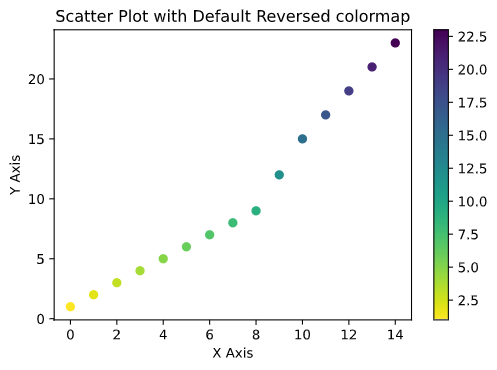In example one, higher values are shown with yellow color and lower values are shown with purple color whereas in example two we can observe that after reversing the colormap higher values are shown with purple color and lower values are shown with yellow color.

Let’s see how to reverse the colormap using the second method which is by using _r at the end of colormap name.

Example 3: Scatter Plot by reversing the colormap by using _r function.

## Python

 `# importing the necessary libraries``import` `numpy as np``import` `matplotlib.pyplot as plt `` ` `# creating the array to plot``x``=``np.arange(``20``)``y``=``[ ``21``, ``24``, ``56``, ``78``, ``43``, ``23``, ``20``, ``28``, ``30``,``   ``4``, ``6``, ``5``, ``7``, ``89``, ``20``, ``12``, ``72``, ``51``, ``58``, ``18``]`` ` `# making the scatter plot on x and y values and giving color w.r.t y``# passing cmap in cmap add _r at the end of colormap name to reverse the colormap``plt.scatter(x, y, c ``=` `y, cmap ``=` `'viridis_r'``)`` ` `# giving name to X and Y axis``plt.xlabel(``"X"``)``plt.ylabel(``"Y"``)`` ` `# visualizing the mapping from values to colors``plt.colorbar()`` ` `# giving title to the plot``plt.title(``"Scatter Plot with Reveresed Viridis colormap"``)`` ` `# visualizing the plot using show() function``plt.show()`

Output: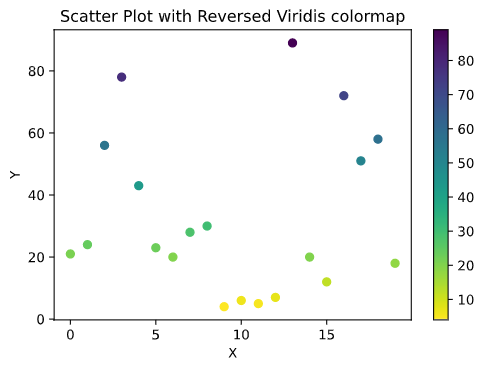Example 4: Scatter Plot with reversed Plasma colormap using Matplotlib.

## Python

 `# importing the necessary libraries``import` `numpy as np``import` `matplotlib.pyplot as plt `` ` `# creating the array to plot``x``=``np.arange(``20``)``y``=``[``21``, ``24``, ``56``, ``78``, ``43``, ``23``, ``20``,``   ``28``, ``30``, ``4``, ``6``, ``5``, ``7``, ``89``, ``20``, ``12``, ``72``,``   ``51``, ``58``, ``18``]`` ` `# making the scatter plot on x and y values and giving color w.r.t y``# passing cmap in cmap add _r at``# the end of colormap name to reverse the colormap``plt.scatter( x, y,c ``=` `y, cmap ``=` `'plasma_r'``)`` ` `# giving name to X and Y axis``plt.xlabel(``"X"``)``plt.ylabel(``"Y"``)`` ` `# visualizing the mapping from values to colors``plt.colorbar()`` ` `# giving title to the plot``plt.title(``"Scatter Plot with Reversed Plasma colormap"``)`` ` `# visualizing the plot using show() function``plt.show()`

Output: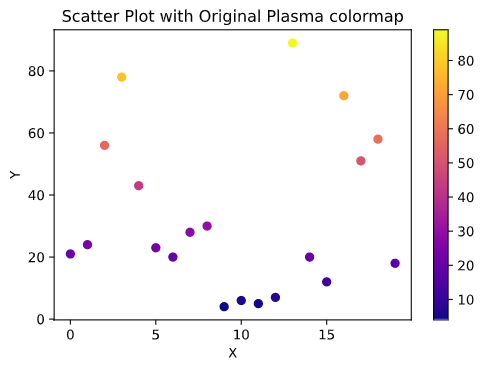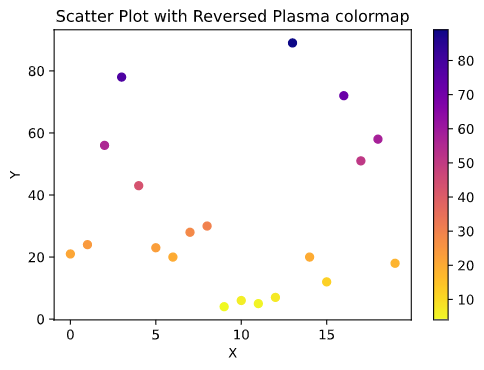In the above example first figure shows the plot without reversing the colormap whereas the second figure shows the plot with reversed colormap. In this first plot higher values are shown with yellow color whereas lower values are shown with dark blue color whereas in the second plot after reversing the colormap higher values are shown with dark blue color and lower values are shown with yellow color. There are many custom colormaps present in matplotlib library like inferno, cividis, magma, plasma, etc.

Attention geek! Strengthen your foundations with the Python Programming Foundation Course and learn the basics.

To begin with, your interview preparations Enhance your Data Structures concepts with the Python DS Course. And to begin with your Machine Learning Journey, join the Machine Learning – Basic Level Course

My Personal Notes arrow_drop_up